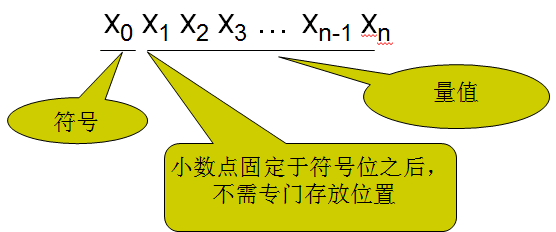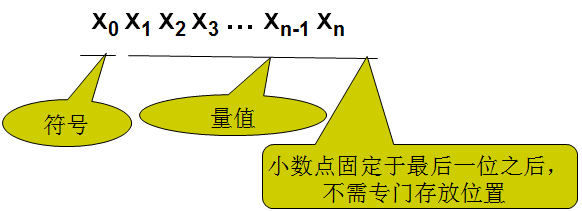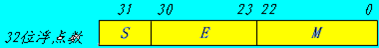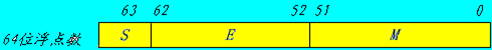# 数据格式(定点表示 浮点表示)

• 定点表示：小数点位置固定(数值范围有限)
• 浮点表示：小数点位置不固定(数值范围很大)

#### 定点表示

• 概念:所有数据的小数点位置固定不变
• 表示方法:符号位+量值(尾数)
• 特点:
数的范围受字长限制，表示数的范围有限;
计算机中多用定点纯整数表示,所以将定点数表示的运算简称为整数运算
• 分类:理论上小数点位置可以任意，但实际上将数据表示成如下两种形式
1. 纯小数(小数点固定在量值最高位的左边,即符号位与量值之间)
表示范围(量值为n位时): $0\le |x|\le 1-{2}^{-n}$$0\leq|x|\leq1-2^{-n}$2. 纯整数(小数点固定在量值最低位的右边)
表示范围(量值为n位时): $0\le |x|\le {2}^{n}-1$$0\leq|x|\leq2^{n}-1$#### 浮点表示

• 概念:小数点位置随阶码不同而浮动
• 表示方法:

• 核心
任意一个二进制数N可以写成 $N={2}^{e}.M$$N=2^e.M$ (如同任意一个十进制数N可以写成$N={10}^{e}.M$$N=10^e.M$ )
其中 $e$$e$ 是浮点数的指数,$M$$M$ 是浮点数的尾数,两者都是有符号的
指数= 阶符+阶码
尾数= 数符+尾数
• 机器中的表示(早期的计算机中)
阶符+阶码+数符+尾数
• IEEE754标准(规定了浮点数的表示格式,运算规则等)
基数2是固定常数,故可不表示出来
尾数用原码,但指数用移码(便于对阶和比较,不需要判断符号位),指数的阶符被隐含在移码里面,故可不表示出来
浮点数的规格化表示: 当尾数的值不为0时,尾数域的最高有效位应为1(类似于0.011强制要求用$1.1\ast {2}^{-2}$$1.1*2^{-2}$表示),又因为最高位固定为1,即尾数域表示的值是1.M,故最高位的1也不予存储.
要除去E用全0和全1表示零和无穷大的情况,如本来是0~255则变成1~254
• 单精度(32位)• S是数的符号位1位，在最高位，“0”表示正数，“1”表示负数
尾数用原码表示,故符号规则同原码
• M尾数23位，在低位部分，采用纯小数表示
注意这里是规格化表示的,即23位字段实际存储24位有效数
• E阶码8位，采用移码表示。
真值e变为阶码E时加上固定的偏移量127,即$E=e+127$$E=e+127$
移码比较大小方便,表示范围从-126~+127移动到1~254
• 双精度(64位)• S是数的符号位1位，在最高位，“0”表示正数，“1”表示负数
尾数用原码表示,故符号规则同原码
• M尾数52位，在低位部分，采用纯小数表示
注意这里是规格化表示的,即52位字段实际存储53位有效数
• E阶码11位，采用移码表示。
真值e变为阶码E时加上固定的偏移量1023,即$E=e+1023$$E=e+1023$
移码比较大小方便,表示范围从-1022~+1023移动到1~2046
• 表示范围

0 0 0 0 0(结合符号位有正零和负零之分)
0 非0 0 非0 正负规格化数
1~254 任意数 1~2046 任意数 正负浮点数
255 0 2047 0 正负无穷
255 非0 2047 非0 不是一个数(NaN)
• 例1(十进制数转浮点数)
十进制$\left(20.59375{\right)}_{10}$$(20.59375)_{10}$数 转换成 754标准的浮点数(32位)的二进制存储格式
1. 分别将整数和分数部分转换成二进制数
$20.59375=10100.10011$$20.59375=10100.10011$
2. 移动小数点，使其在第1，2位之间(格式化浮点数,小数点在最高位的1后)
$10100.10011=1.010010011×{2}^{4}$$10100.10011=1.010010011×2^4$
3. 由真值e加偏移量(32位为127,64位为1023)得阶码E
$E=e+127=4+127=131=10000011$$E=e+127=4+127=131=10000011$
$S=0$$S=0$
$M=010010011$$M=010010011$
4. 得32位浮点数的二进制存储格式(不满32位后面要补0)
0100 0001 1010 0100 1100 0000 0000 0000=$\left(41A4C000{\right)}_{16}$$(41A4C000)_{16}$
• 例2(浮点数转十进制数)
754标准存储格式为$\left(41360000{\right)}_{16}$$(41360000)_{16}$的浮点数 转 十进制数
1. 将数展开,用二进制格式表示
得,0 100 00010 011 0110 0000 0000 0000 0000
2. 根据对应位置,得
$S=0$$S=0$
$e=E-127=10000010-01111111=00000011=\left(3{\right)}_{10}$$e=E-127=10000010-01111111=00000011=(3)_{10}$
尾数补回隐藏位1,得
$1.M=1.01101100000000000000000=1.011011$$1.M=1.011 0110 0000 0000 0000 0000=1.011011$
故十进制数为
$\left(-1{\right)}^{s}×1.M×{2}^{e}=+\left(1.011011\right)×{2}^{3}=+1011.011=\left(11.375{\right)}_{10}$$(-1)^s×1.M×2^e=+(1.011011)×2^3=+1011.011=(11.375)_{10}$